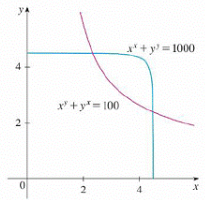Chapter 14, Problem 6P

Chapter
Section
Textbook Problem

(a) Newton's method for approximating a root of an equation f(x) = 0 (see Section 4.8) can be adapted lo approximating a solution of a system of equations f(x, y) = 0 and g(x, y) = 0. The surfaces z = f(x, y) and z = g(x, y) intersect in a curve that intersects the xy-plane at the point (r, s), which is the solution of the system. If an initial approximation (x1, y1) is close to this point, then the tangent planes to the surfaces at (x1, y1) intersect in a straight line that intersects the xy-plane in a point (x2, y2), which should be closer to (r, s). (Compare with Figure 4.8.2.) Show that x 2 = x 1 − f g y − f y g f x g y − f y g x and y 2 = y 1 − f x g − f g x f x g y − f y g x where f, g, and their partial derivatives are evaluated at (x1, y1). If we continue this procedure, we obtain successive approximations (xn, yn).(b) It was Thomas Simpson (1710–1761) who formulated Newton's method as we know it today and who extended it to functions of two variables as in part (a). (See the biography of Simpson on page 520.) The example that he gave to illustrate the method was to solve the system of equationsxx + yy= 1000 xy + yx= 100In other words, he found the points of intersection of the curves in the figure. Use the method of part (a) to find the coordinates of the points of intersection correct to six decimal places.(a)

To determine

To show: The equations x2=x1fgyfygfxgyfygx and y2=y1fxgfgxfxgyfygx and their partial derivatives f , g are evaluated at (x1,y1) .

Explanation

Given:

Equation of x2 is x2=x1fgyfygfxgyfygx .

Equation of y2 is y2=y1fxgfgxfxgyfygx .

The surface equations which intersect in a curve are z=f(x,y) and z=g(x,y) .

Calculation:

At the point (x1,y1,0) the equation of the tangent plane (P1) to z=f(x,y) is as below,

zf(x1,y1)=fx(x1,y1)(xx1)+fy(x1,y1)(yy1)

Write the equation of the tangent plane (P2) to z=g(x,y) is as below,

zg(x1,y1)=gx(x1,y1)(xx1)+gy(x1,y1)(yy1)

When z=0 , the equation of the xy plane intersected by P1 is

f(x1,y1)=fx(x1,y1)(xx1)+fy(x1,y1)(yy1) (1).

When z=0 , the equation of the xy plane intersected by P2 is

g(x1,y1)=gx(x1,y1)(xx1)+gy(x1,y1)(yy1) (2).

At the point (x2,y2,0) the line of intersection of two tangent planes intersecting xy plane is determined as,

f(x1,y1)=fx(x1,y1)(x2x1)+fy(x1,y1)(y2y1) (3).

At the point (x2,y2,0) the line of intersection of two tangent planes intersecting xy plane is determined as,

g(x1,y1)=gx(x1,y1)(x2x1)+gy(x1,y1)(y2y1) (4).

Substitute fx for fx(x1,y1) , fy for fy(x1,y1) , gx for gx(x1,y1) , gy for gy(x1,y1) , f for f(x1,y1) and g for g(x1,y1) and substitute in equation (1),

f(x1,y1)=fx(x1,y1)(xx1)+fy(x1,y1)(yy1)f=fx(xx1)+fy(yy1) (5).

Substitute fx for fx(x1,y1) , fy for fy(x1,y1) , gx for gx(x1,y1) , gy for gy(x1,y1) , f for f(x1,y1) and g for g(x1,y1) and substitute in equation (2),

g(x1,y1)=gx(x1,y1)(xx1)+gy(x1,y1)(yy1)g=gx(xx1)+gy(yy1) (6).

Substitute fx for fx(x1,y1) , fy for fy(x1,y1) , gx for gx(x1,y1) , gy for gy(x1,y1) , f for f(x1,y1) and g for g(x1,y1) and substitute in equation (3),

f(x1,y1)=fx(x1,y1)(x2x1)+fy(x1,y1)(y2y1)f=fx(x2x1)+fy(y2y1) (7).

Substitute fx for fx(x1,y1) , fy for fy(x1,y1) , gx for gx(x1,y1) , gy for gy(x1,y1) , f for f(x1,y1) and g for g(x1,y1) and substitute in equation (4),

g(x1,y1)=gx(x1,y1)(x2x1)+gy(x1,y1)(y2y1)g=gx(x2x1)+gy(y2y1) (8)

(b)

To determine

To find: The coordinates of the point of intersection and correct to the six decimal places.

Still sussing out bartleby?

Check out a sample textbook solution.

See a sample solution

The Solution to Your Study Problems

Bartleby provides explanations to thousands of textbook problems written by our experts, many with advanced degrees!

Get Started

Given f(x)=x2+3, evaluate each expression. f(x+x) b. f(x+x)f(x)x

Calculus: An Applied Approach (MindTap Course List)

Solve each equation and check: 5m=0

Elementary Technical Mathematics

True or False: If f(x) = F(x), then baf(x)dx=F(b)F(a).

Study Guide for Stewart's Single Variable Calculus: Early Transcendentals, 8th